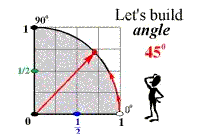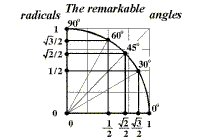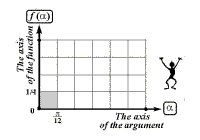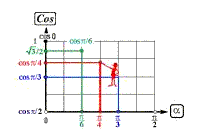The main -> Visual didactic materials -> Mathematics ->Trigonometry-> The first quarter of the trigonometrical circumference
 Visual didactic materials «The first quarter of the trigonometrical circumference»            The series «The first quarter of the trigonometrical circumference» of the collection «The trigonometrical circumference» is aimed to form primary ideas of the most important trigonometrical concepts.            The main aim is for pupils to see what «remarkable numbers» they can see, how and where, going to construction and visual determination of correlation of sines and cosines of the remarkable angles to these numbers then; for them to find out what part the «remarkable points» play in constructionof the graph of function onto the interval from 0 to П/2.            These films can be reproduced on any computer having WINDOWS version 95 (and higher versions) without an additional installation of special programmes as they are made in EXE format.            The principle of operation: the changing of frames is executed by pushing of a printed(graphical) button. Movement is is possible both “forward” and “backward”.            Slide-films are meant for group study and can be used by a teacher at school lessons spontaneity.The principle of operation the film offering by us gives an opportunity to stop at the frames demanding special attention.

 №1. The remarkable angles of the first quarter of the trigonometrical circumferenceThe film shows how to construct remarkable angles rather exactly without a protractoron by means of a pen and a pair of compasses on plotted paper .        It’s useful to pay attention of students to comfortable for calculations and corresponding constructions significances of the remarkable angles, for which angles of 0, 30, 45, 60 and 90 degrees of the first quarter of the trigonometrical circumference are taken. Slide-film viewing

 №2. The remarkable radicals on the radiuses of the first quarter of the trigonometrical circumferenсeThe film shows how to mark some special numbers which are called the remarkable radicals on the radiuses of the trigonometricalcircumferenсe there rather precise on plotted paper.        It’s useful to pay attention of students to comfortable for calculations and corresponding constructions significances of the remarkable radicals, which are the coordinates of the points forming on the first quarter of the trigonometrical circumference. Slide-film viewing

 №3. The remarkable sines and cosines of the angles of the first quarter of the trigonometrical circumferenceThe film shows how to determine the significances of sines and cosines of the remarkable angles on the base of already learnt ways        The connection of the remarkable angles of the first quarter of the trigonometrical circumference and corresponding remarkable sines and cosines as which sines and cosines of the remarkable angles are implied should be paid attention of students to. Slide-film viewing

 №4. The radian measure of the remarkable angles of the first quarter of the trigonometrical circumferenceThe film shows how to mark sines and cosines of the remarkable angles of the first quarter of the trigonometrical circumference expressed in radians on the line of sines and cosines. Besides,the comparison of equal significances of remarkable sines and cosines for different angles takes place.        The way the radian and the degree measures of the remarkable angles are connected should be paid attention to.

 №5. The remarkable radians and numbers of the first quarter of the trigonometrical circumferenceThe film shows how to determine rather exactly the position of the remarkable radians and radicals on the axis of special trigonometrical system of coordinates hith help of of the models of the first quarter of the trigonometrical circumferenсe there on plotted paper. Slide-film viewing

 №6. The graph of sine in the interval from 0 to П/2 on the plotted paperThe film shows how to construct the graph of sines and cosines in the interval from 0 to П/2 with help of the remarkable points of the first quarter of the trigonometrical circumferenсe on plotted paper.        The students are supposed to be given the basic ideas of construction of the graph of sines in the trigonometrical co-ordinate system, which is determined as a curve passing through the remarkable points of the sine. Slide-film viewing

 №7. The graph of cosine in the interval from 0 to П/2 on the plotted paperThe film shows that construction of the remarkable cosines on plotted paper also takes place on the axis of significance of the trigonometrical function.The remarkable radians are also laid of the first quarter of the trigonometrical circumferenсe on the axis of its argument.        The students are supposed to be given the basic ideas of peculiarities of construction of the graph of cosines in the trigonometrical system of coordinates, which is also determined as a curve passing through its remarkable points . Slide-film viewing
 The author’s group: Conception and visualisation - Reznik N.A. Planning and visual organisation - Pavlov N.A. Selection of lexical material,approving and adaptation - Karasev A.A. Mathematical editing and тesting - Temnikova I.S.
 The main -> Visual didactic materials -> Mathematics-> Trigonometry-> The first quarter of the trigonometrical circumferenсe

Visual lessons | Visual didactic materials | Our publications | About us | Reviews | News of the site | Contacts |Our friends

 © Natalya Reznik (nareznik@yandex.ru): the chief of the project “The visual school” © Pavlov Nikolay (pavlov.nikolay@gmail.com): the methodologist-elaborator of the web site © Aleksey Baryshkin: design the mock-up of the web site © Anrey Karasev: translator